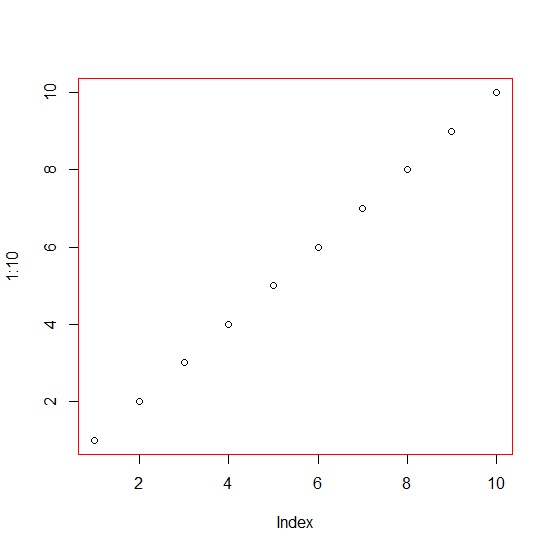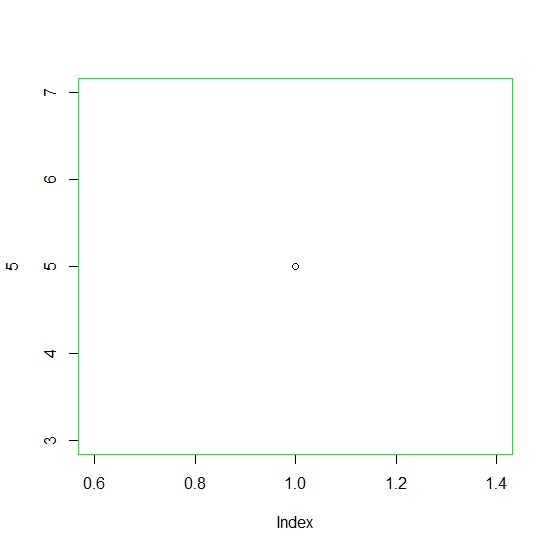# How to change the border color of box of a base R plot?

To change the border color of box of a base R plot, we can use box function with col argument where we can pass the color other than black because black is the default color. We first need to create the plot using plot function and then box function will be used as shown in the below examples.

## Example 1

To change the border color of box of a base R plot, use the command given below −

plot(1:10)
box(col="red")

## Output

If you execute the above given command, it generates the following output −## Example 2

To change the border color of box of a base R plot, use the command given below −

plot(5)
box(col="green")

## Output

If you execute the above given command, it generates the following output −